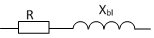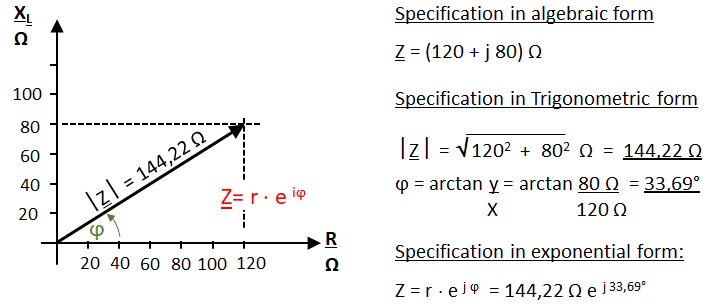# Complex numbers in electrical engineering

(Deutsch)Learnchannel Nachhilfe

### Complex numbers in electrical engineering

In mathematics, it is common to denote the imaginary part with the letter i. In electrical engineering, however, the letter i is reserved for the electric current. To avoid confusion, we use j for the imaginary part in the following: Z = x + j y

Examples for the application of the complex numbers in electrical engineering now in the following:

Example 1: Series connection of R and XlSeries connection of ohmic and inductive resistance

What you see is a series connection of an ohmic resistor of 120 Ω and an inductive resistor XL of 80 Ω. Determine mathematically and graphically the complex resistance Z of the series circuit.

Solution:Complex numbers applied to a series connection of R and XL

... coming soon more